# Using the Symbol Library

The Symbol Library lets you select multiple symbols from all the symbols provided by the SOLIDWORKS software. You can also use the Windows Character Map to select additional symbols to insert.

SOLIDWORKS provides symbol categories for specific types of annotation, such as weld symbols, geometric tolerancing symbols, and modifying symbols.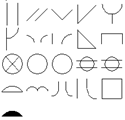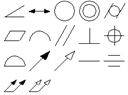ANSI Weld Symbols Geometric Tolerancing Symbols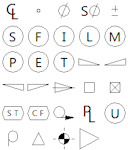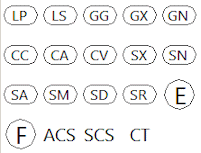Modifying Symbols ISO Tolerancing Symbols

Symbols categories:

Category Example Description
ANSI Lay Symbols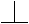Perpendicularity
Weld Contour Symbols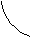Fillet with concave
Weld Spacer Symbols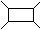Double V groove spacer
Weld Symbols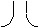Flange edge
Draft Draft Symbols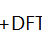Plus Draft
Flag Circle 1-99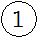Circle 1
Circle 1.-99.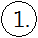Circle 1.
Circle A-Z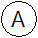Circle A
Circle A.-Z.Circle A.
Square 1-99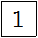Square 1
Square 1.-99.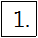Square 1.
Square A-Z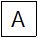Square A
Square A.-Z.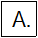Square A.
Square Circle 1-99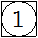Square/Circle 1
Square Circle 1.-99.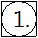Square/Circle 1.
Square Circle A-Z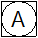Square/Circle A
Square Circle A.-Z.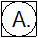Square/Circle A.
Triangle 1-99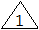Triangle 1
Triangle 1.-99.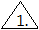Triangle 1.
Triangle A-Z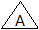Triangle A
Triangle A.-Z.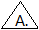Triangle A.
Triangle Rotated 1-99Triangle-rotated 1
Triangle Rotated 1.-99.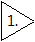Triangle-rotated 1.
Triangle Rotated A-Z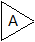Triangle-rotated A
Triangle Rotated A.-Z.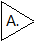Triangle-rotated A.
Geometric Tolerancing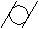Cylindricity
GOST Geometric Tolerancing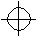Position
Weld Symbols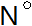Number (No)
Hole Symbols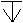Depth/Deep
Inspection Symbols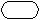Inspection box (empty)
ISO Geometric Tolerancing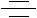Symmetry
Tolerancing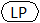Two-point size
Weld Contour Symbols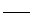Flat
Weld Symbols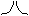Butt
JIS Weld Symbols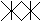JIS seam
Key Symbol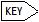Key
Modifying Symbols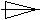Conical taper
View Symbols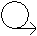GOST unfold